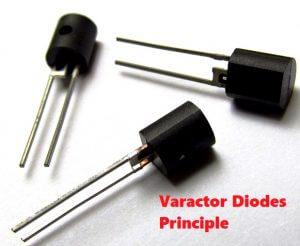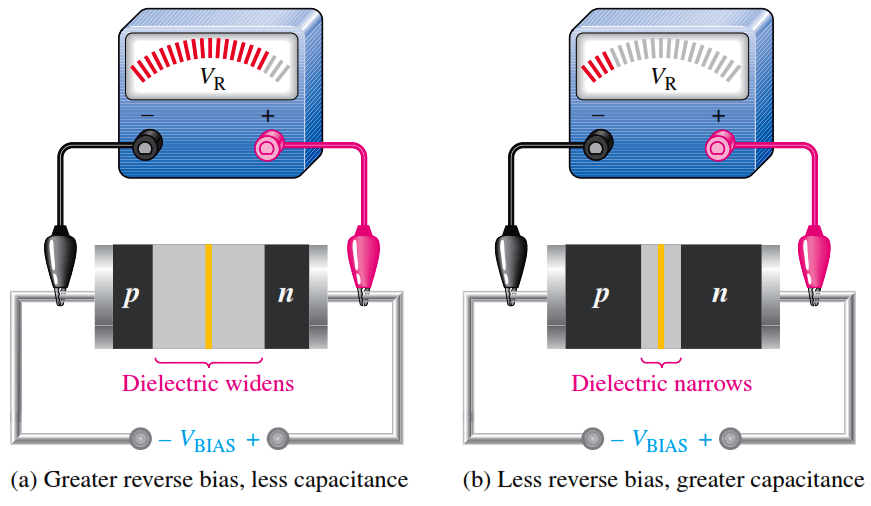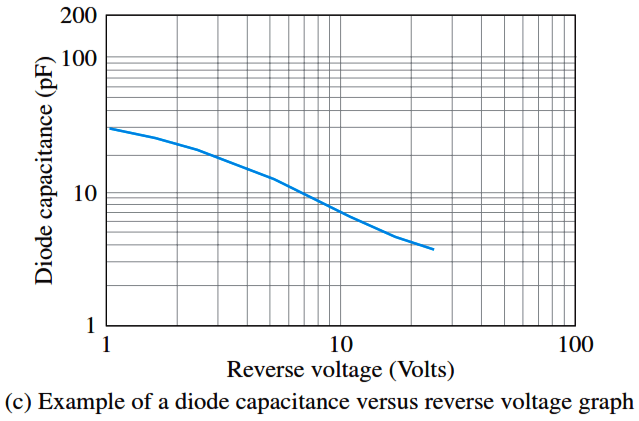# Varactor Diode Operation

The junction capacitance of diodes varies with the amount of reverse bias. Varactor diodes are specially designed to take advantage of this characteristic and are used as Voltage Controlled capacitors rather than traditional diodes.These devices are commonly used in communication systems. Varactor diodes are also referred to as varicaps or tuning diodes.A varactor is a diode that always operates in reverse bias and is doped to maximize the inherent capacitance of the depletion region. The depletion region acts as a capacitor dielectric because of its nonconductive characteristic. The p and n regions are conductive and act as the capacitor plates, as illustrated in Figure.Fig : The reverse-biased varactor diode acts as a variable capacitor.

Basic Operation

Capacitance is determined by the parameters of plate area (A), dielectric constant (P), and plate separation (d), as expressed in the following formula:As the reverse-bias voltage increases, the depletion region widens, effectively increasing the plate separation, thus decreasing the capacitance. When the reverse-bias voltage decreases, the depletion region narrows, thus increasing the capacitance. This action is shown in Figure (a) and (b). A graph of diode capacitance (CT) versus reverse voltage for a certain varactor is shown in Figure (c). For this particular device, CT varies from 30 pF to slightly less than 4 pF as VR varies from 1 V to 30 V.In a varactor diode, these capacitance parameters are controlled by the method of doping near the pn junction and the size and geometry of the diode’s construction. Nominal varactor capacitances are typically available from a few picofarads to several hundred picofarads.Fig : Varactor diode capacitance varies with reverse voltage.

Applications

A major application of varactors is in tuning circuits. For example, VHF, UHF, and satellite receivers utilize varactors. Varactors are also used in cellular communications.